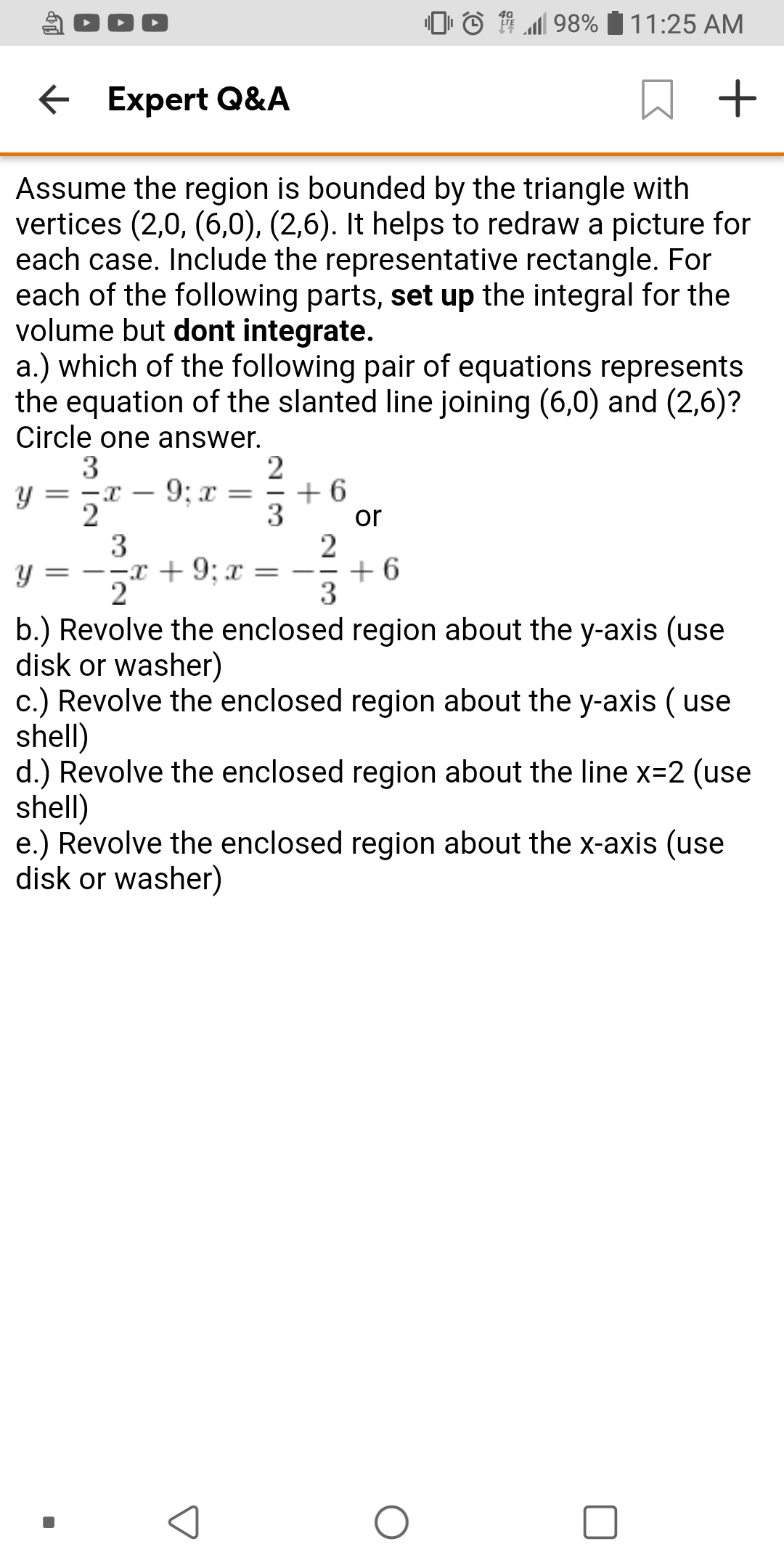98% 11:25 AMExpert Q&AAssume the region is bounded by the triangle withvertices (2,0, (6,0), (2,6). It helps to redraw a picture foreach case. Include the representative rectangle. Foreach of the following parts, set up the integral for thevolume but dont integrate.a.) which of the following pair of equations representsthe equation of the slanted line joining (6,0) and (2,6)?Circle one answer.3y =263269; x-2or3=--x + 9; x =y2b.) Revolve the enclosed region about the y-axis (usedisk or washer)c.) Revolve the enclosed region about the y-axis ( useshell)d.) Revolve the enclosed region about the line x-2 (useshell)e.) Revolve the enclosed region about the x-axis (usedisk or washer)

Question

Part d and ehelp_outlineImage Transcriptionclose98% 11:25 AM Expert Q&A Assume the region is bounded by the triangle with vertices (2,0, (6,0), (2,6). It helps to redraw a picture for each case. Include the representative rectangle. For each of the following parts, set up the integral for the volume but dont integrate. a.) which of the following pair of equations represents the equation of the slanted line joining (6,0) and (2,6)? Circle one answer. 3 y = 2 6 3 2 6 9; x - 2 or 3 =--x + 9; x = y 2 b.) Revolve the enclosed region about the y-axis (use disk or washer) c.) Revolve the enclosed region about the y-axis ( use shell) d.) Revolve the enclosed region about the line x-2 (use shell) e.) Revolve the enclosed region about the x-axis (use disk or washer) fullscreen
Step 1

(D) When the enclosed region revolve about x=2 line then the radius is on the line joining (2,0) and (6,0).

And the height is on the line joining (2,6),(6,0).

Step 2

And to find the height we need the equation of the line joining (2,6),(6,0). That is y=(-3/2)x+9

Step 3

Answer (D): That is the required...

Want to see the full answer?

See Solution

Want to see this answer and more?

Our solutions are written by experts, many with advanced degrees, and available 24/7

See Solution
Tagged in

Integration